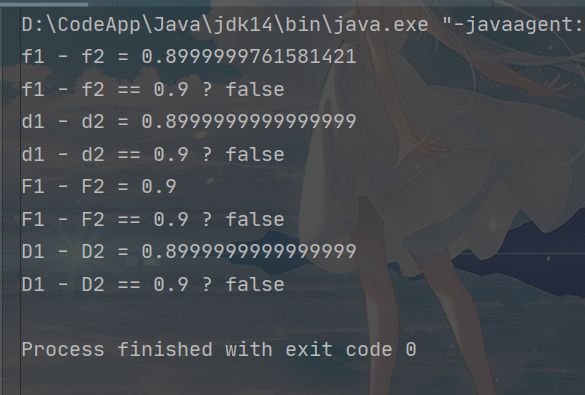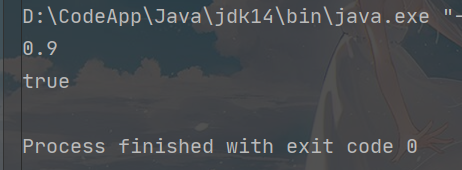# 前言

``````public class Main {
public static void main(String[] args) {
float f1 = 2.0f;
float f2 = 1.1f;
System.out.println("f1 - f2 = " + (double)(f1 - f2));
System.out.println("f1 - f2 == 0.9 ? " + (f1 - f2 == 0.9));

double d1 = 2.0;
double d2 = 1.1;
System.out.println("d1 - d2 = " + (d1 - d2));
System.out.println("d1 - d2 == 0.9 ? " + (d1 - d2 == 0.9));

Float F1 = 2.0f;
Float F2 = 1.1f;
System.out.println("F1 - F2 = " + (F1 - F2));
System.out.println("F1 - F2 == 0.9 ? " + (F1 - F2 == 0.9));

Double D1 = 2.0;
Double D2 = 1.1;
System.out.println("D1 - D2 = " + (D1 - D2));
System.out.println("D1 - D2 == 0.9 ? " + (D1 - D2 == 0.9));
}
}
``````# BigDecimal

## 使用BigDecimal进行`2.0-1.1`运算

``````public class Main {
public static void main(String[] args) {
BigDecimal b1 = BigDecimal.valueOf(2.0);
BigDecimal b2 = BigDecimal.valueOf(1.1);
System.out.println(b1.subtract(b2).doubleValue());
System.out.println(0.9 == b1.subtract(b2).doubleValue());
}
}
``````# BigDecimal常用方法

## BigDecimal() 构造方法

• subtract方法，进行减法运算，减去传入的BigDecimal对象。
• multiply方法，进行乘法运算，乘以传入的BigDecimal对象。
• divide方法，进行除法运算，除以传入的BigDecimal对象。

## toString、doubleValue、floatValue、longValue、intValue

• toString方法，将BigDecimal对象的值转换成一个字符串对象并返回。
• doubleValue方法，将BigDecimal对象的值转换成一个双精度浮点型数并返回。
• floatValue方法，将BigDecimal对象的值转换成一个单精度浮点型数并返回。
• longValue方法，将BigDecimal对象的值转换成一个长整数并返回。
• intValue方法，将BigDecimal对象的值转换成一个整数并返回。

## compareTo方法比较

• a = -1,表示bigdemical小于bigdemical2；
• a = 0,表示bigdemical等于bigdemical2；
• a = 1,表示bigdemical大于bigdemical2；

# BigDecimal常见异常

`int a = bigdemical.divide(bigdemical2,2)`，此时，在进行bigdemical/bigdemical2时，就会只保留两位小数。

Q.E.D.

••